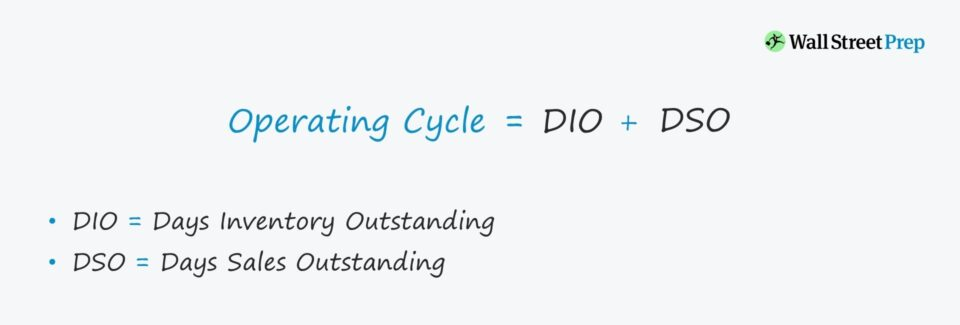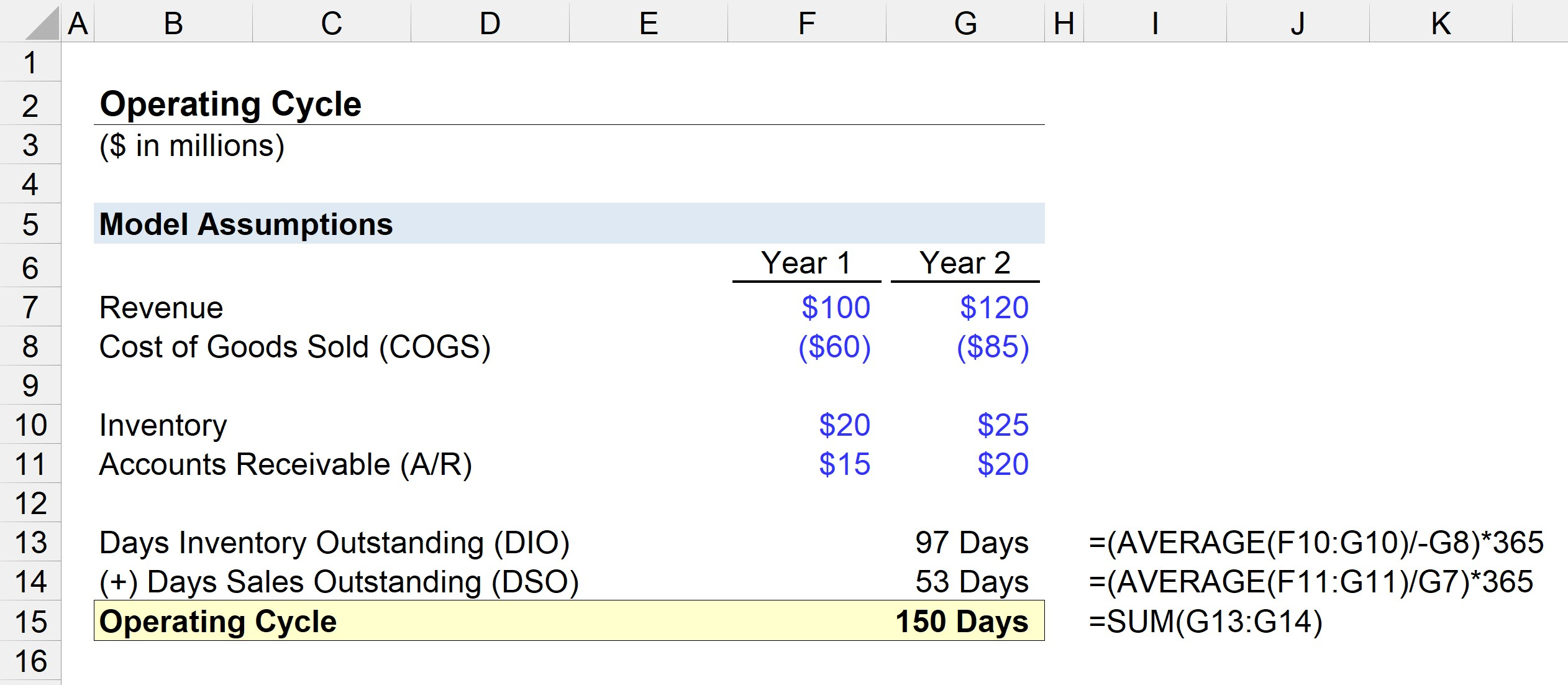Welcome to Wall Street Prep! Use code at checkout for 15% off.# Operating Cycle

Guide to Understanding the Operating Cycle## How to Calculate the Operating Cycle

Conceptually, the operating cycle measures the time it takes a company on average to purchase inventory, sell the finished inventory, and collect cash from customers that paid on credit.

• Start of Cycle: The “start” of the cycle refers to the date when the inventory (i.e. raw material) was purchased by the company to turn it into a marketable product available for sale.
• End of Cycle: The”end” is when cash payment for the product purchase is received from customers, who often pay on credit as opposed to cash (i.e. accounts receivable).

The required inputs for the metric consist of two working capital metrics:

###### Formula

Below are the formulas for calculating the two working capital metrics:

• DIO = (Average Inventory / Cost of Goods Sold) * 365 Days
• DSO = (Average Accounts Receivable / Revenue) * 365 Days

## Operating Cycle Formula

The formula for calculating the operating cycle is as follows.

###### Formula
• Operating Cycle = DIO + DSO

The calculation of the operating cycle is relatively straightforward, but more insights can be derived from examining the drivers behind DIO and DSO.

For instance, the duration of a particular company could be high relative to that of comparable peers. Such an issue could stem from the inefficient collection of credit purchases, rather than being due to supply chain or inventory turnover issues.

Once the real underlying issue has been identified, management can better address and fix the problem.

## How to Interpret the Operating Cycle

The longer the operating cycle, the more cash is tied up in operations (i.e. working capital needs), which directly lowers a company’s free cash flow (FCF).

• Lower: The company’s operations are more efficient – all else being equal.
• Higher: On the other hand, higher operating cycles point towards weaknesses in the business model that must be addressed.

## Operating Cycle vs. Cash Conversion Cycle

The cash conversion cycle (CCC) measures the number of days for a company to clear out its inventory in storage, collect outstanding A/R in cash, and delay payments (i.e. accounts payable) owed to suppliers for goods/services already received.

###### Formula
• Cash Conversion Cycle (CCC) = Days Inventory Outstanding (DIO) + Days Sales Outstanding (DSO) – Days Payable Outstanding (DPO)

At the start of the calculation, the sum of DIO and DSO represents the operating cycle – and the added step is subtracting DPO.

Hence, the cash conversion cycle is used interchangeably with the term “net operating cycle”.

## Operating Cycle Calculator – Excel Template

We’ll now move to a modeling exercise, which you can access by filling out the form below.Submitting...

## Operating Cycle Example Calculation

Suppose we’re tasked with assessing the working capital efficiency of a company with the following assumptions:

Year 1 Financials

Year 2 Financials

• Revenue: \$120 million
• Cost of Goods (COGS): \$85 million
• Inventory: \$25 million
• Accounts Receivable (A/R): \$20 million

The first step is to calculate DIO by dividing the average inventory balance by the current period COGS and then multiplying it by 365.

• DIO = AVERAGE (\$20m, \$25m) / \$85 * 365 Days
• DIO = 97 Days

On average, it takes the company 97 days to purchase raw material, turn the inventory into marketable products, and sell it to customers.

In the next step, we will calculate DSO by dividing the average A/R balance by the current period revenue and multiplying it by 365.

• DSO = AVERAGE (\$15m, \$20m) / \$120m * 365 Days
• DSO = 53 Days

The operating cycle is equal to the sum of DIO and DSO, which comes out to 150 days in our modeling exercise.

• Operating Cycle = 97 Days + 53 Days = 150 DaysStep-by-Step Online Course

### Everything You Need To Master Financial Modeling

Enroll in The Premium Package: Learn Financial Statement Modeling, DCF, M&A, LBO and Comps. The same training program used at top investment banks.Inline FeedbacksLearn Financial Modeling Online

Everything you need to master financial and valuation modeling: 3-Statement Modeling, DCF, Comps, M&A and LBO.

X

The Wall Street Prep Quicklesson Series

7 Free Financial Modeling Lessons

Get instant access to video lessons taught by experienced investment bankers. Learn financial statement modeling, DCF, M&A, LBO, Comps and Excel shortcuts.